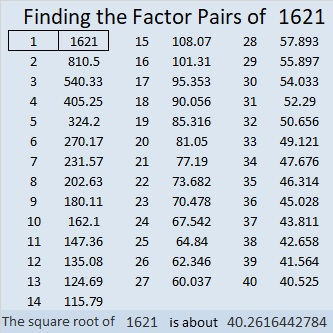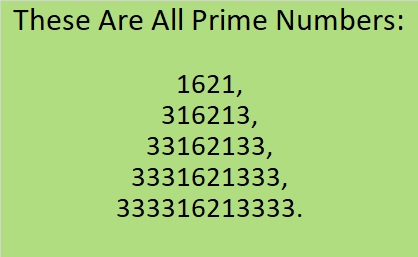1621 Give This Purple Egg a Crack!

Contents

Today’s Puzzle:

Here’s a level-5 purple Easter egg for you to try. All you need to do is write the numbers from 1 to 12 in both the first column and in the top row so that those numbers and the given clues function like a multiplication table. Go ahead. Give it a crack!Factors of 1621:

• 1621 is a prime number.
• Prime factorization: 1621 is prime.
• 1621 has no exponents greater than 1 in its prime factorization, so √1621 cannot be simplified.
• The exponent in the prime factorization is 1. Adding one to that exponent we get (1 + 1) = 2. Therefore 1621 has exactly 2 factors.
• The factors of 1621 are outlined with their factor pair partners in the graphic below.How do we know that 1621 is a prime number? If 1621 were not a prime number, then it would be divisible by at least one prime number less than or equal to √1621. Since 1621 cannot be divided evenly by 2, 3, 5, 7, 11, 13, 17, 19, 23, 29, 31, or 37, we know that 1621 is a prime number.

OEIS.org informs us that 1621 is in an interesting group of prime numbers.I have verified it. They really are all prime!

1621 is the sum of two squares:
39² + 10² = 1621.

1621 is the hypotenuse of a Pythagorean triple:
780-1421-1621, calculated from 2(39)(10), 39² – 10², 39² + 10².

Here’s another way we know that 1621 is a prime number: Since its last two digits divided by 4 leave a remainder of 1, and 39² + 10² = 1621 with 39 and 10 having no common prime factors, 1621 will be prime unless it is divisible by a prime number Pythagorean triple hypotenuse less than or equal to √1621. Since 1621 is not divisible by 5, 13, 17, 29, or 37, we know that 1621 is a prime number.

This site uses Akismet to reduce spam. Learn how your comment data is processed.